# 1 Introduction

Panta Rhei; everything flows.

‘PantaRhei’ is an R package to produce Sankey diagrams. Sankey diagrams visualize the flow of conservative substances through a system. They typically consists of a network of nodes, and fluxes between them, where the total balance in each internal node is 0, i.e. input equals output. Sankey diagrams differ from so-called alluvial diagrams because they allow for cyclic flows: flows originating from a single node can, either direct or indirect, contribute to the input of that same node. Sankey diagrams are typically used to display energy systems, material flow accounts etc. ‘PantaRhei’ employs a simple syntax to set up diagrams using data in tables, such as spread sheets. ‘PantaRhei’ is capable to produce publication-quality diagrams.

As an example of the power of ‘PantaRhei’, consider the next example, based on data from Statistics Netherlands and an original diagram design by Haas et al, (2005))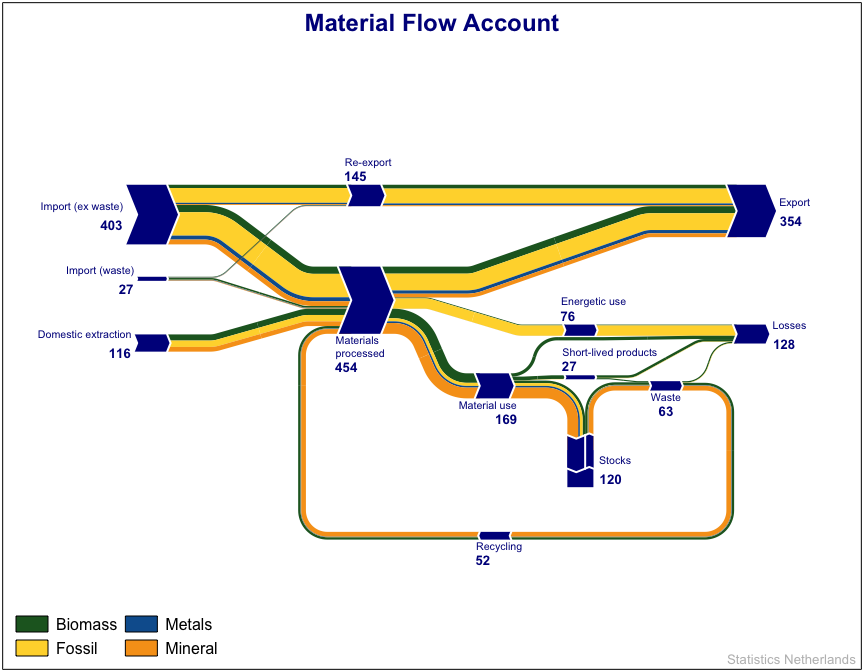Don’t get intimidated by this example. We will start gently.

# 2 A Simple example.

To create a Sankey diagram, you’ll need three different data frames, providing information on

• nodes
• flows
• colors

The nodes data frame provides information on the nodes: at least an unique identifier and their position.

• ID (character) to identify the node
• x (numeric) the x-coordinate of the node (in arbitrarly units)
• y (numeric) the y-coordinate of the node.

There are some additional fields, but these are optional, and will be described later.

Let’s start with a simple example; using two nodes A and B. The data frame can be set up as follows:

nodes <- data.frame(
ID =c("A", "B"),
x  = c(1, 2),
y  = c(0, 0)
)

note For real-world applications, data are likely read from Excel spreadsheets or similar; look at the end of this manual to see some examples.

ID x y
A 1 0
B 2 0

The flows data frame provides information on the flow between the nodes. it requires at minimum

• from (character) the ID of the starting node
• to (character) the ID of the ending node
• quantity (numeric) the magnitude of the flow.
flows <- data.frame(
from      = "A",
to        = "B",
quantity  = 10.0
)
from to quantity
A B 10

A Sankey diagram is then produced by calling

sankey(nodes, flows)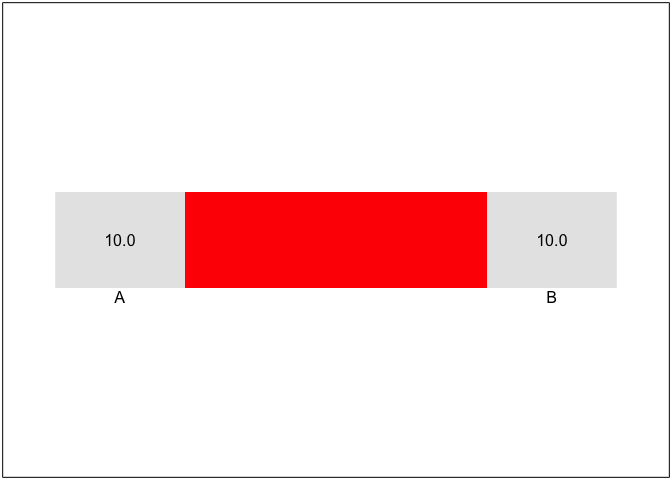Note the following:

• The two nodes A and B are plotted next to each other. The coordinates (1,0) and (2,0) are scaled such that the diagram takes up the whole plot area (minus a margin)
• Node IDs are plotted below the nodes
• For each node, the total flow that passes through that node is accumulated (the node magnitude), and plotted in the node.
• An automatic color has been chosen.

# 3 A simple material flow.

This example is a bit more complex We introduce the following extensions:

• More nodes
• Node labels, and node placement.
• legends

It is often useful to have node labels that are descriptive, or to have labels that are in a different language. To this end, a character column label is available. Note that by default (as in example 1) the node ID is used as label.

It is also useful to have some control on label placement. This can be specified by the column label_pos which accepts the values left, right, above and below, which act as expected.

The following example specifies 4 nodes for a highly stylized material flow diagram.

nodes <- tribble(
~ID,    ~label,          ~x, ~y, ~label_pos,
"imp",  "Import",         1,  2, "left",
"exp",  "Export",         5,  2, "right",
"dom",  "Domestic use",   5,  1, "above",
"proc", "Processing",     3,  1, "below"
)
ID label x y label_pos
imp Import 1 2 left
exp Export 5 2 right
dom Domestic use 5 1 above
proc Processing 3 1 below

It is also useful to have multiple flow types, or substances, representing for instance different materials, such as biotic and mineral, or different energy carriers, such as oil, gas, coal and electricity, or different food commodities, as in the next example.

flows <- tribble(
~from,  ~to,   ~substance, ~quantity,
"imp",  "exp", "Cocoa",     10,
"imp",  "proc", "",          5,
"proc", "dom",  "",          2,
"proc", "exp",  "",          3,
"imp",  "exp",  "Sugar",     2,
"imp",  "proc", "",          6,
"proc", "dom",  "",          5,
"proc", "exp",  "",          1
)
from to substance quantity
imp exp Cocoa 10
imp proc 5
proc dom 2
proc exp 3
imp exp Sugar 2
imp proc 6
proc dom 5
proc exp 1

Note there that it is not required to repeat the substance labels for every row in the table. For rows where it is left blank, the last specified value is re-used.

The following example uses these nodes and flows to draw a simplified material flow Sankey diagram. By adding the option legend=TRUE a legend is included.

sankey(nodes,flows, legend=TRUE)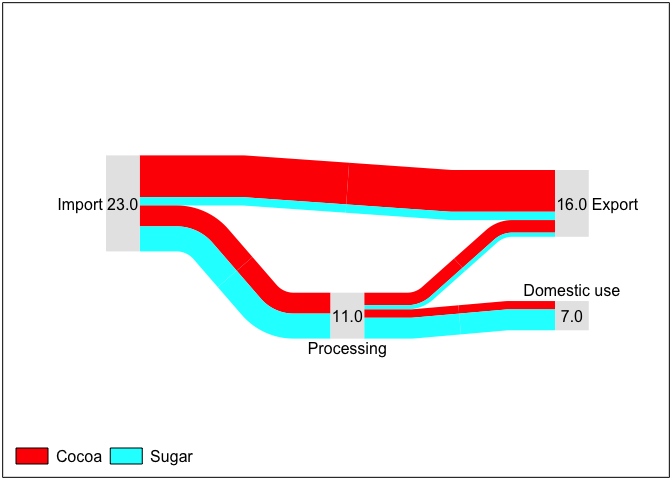## 3.1 Specifying flow colors

In the previous example, colors for the various flowing substances, in this example cocoa and sugar, were defined automatically (to be precise: using the rainbow() function of base R).

Colors can be specified by using a separate ‘colors’ data frame:

colors <- tribble(
~substance, ~color,
"Cocoa",    "chocolate",
"Sugar",    "#FFE4C4"
)
substance color
Cocoa chocolate
Sugar #FFE4C4

Note that all color specifications that R understands are allowed. For example, red can be specified by "red", "#FF00000" and rgb(1,0,0). (use colors() or search the internet for R colors to learn more about R color names)

sankey(nodes, flows, colors, legend=TRUE)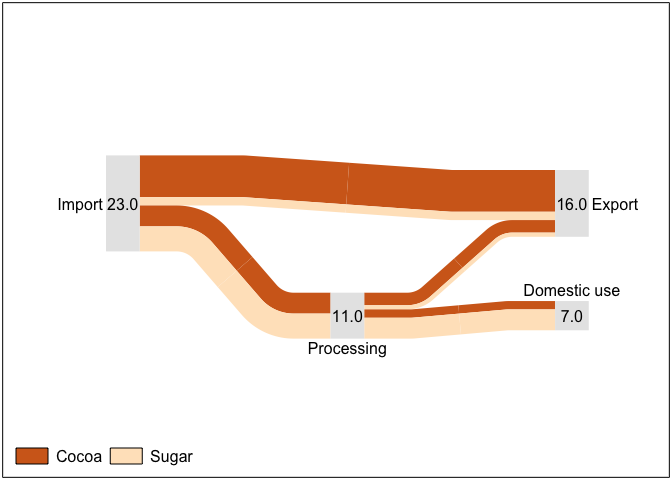## 3.2 Node placement

Node locations can be specified relative to each other. In the next example the ‘Domestic use’ node is placed at the same x-coordinate as the Export node, by using the relative x-coordinate "exp"

nodes <- tribble(
~ID,    ~label,          ~x, ~y, ~label_pos,
"imp",  "Import",         "1",   2,   "left",
"exp",  "Export",         "5",   2,   "right",
"dom",  "Domestic use",   "exp", 1,  "above",
"proc", "Processing",     "3",   1,   "below"
)
sankey(nodes, flows, colors, legend=TRUE)Note that we could also place the nodes at a certain distance, e.g. by specifying exp+1 to ensure that node dom is always 1 unit to the right of node exp.

Also note that while the Export node is at the same y-coordinate as Import, the flow between them looks crooked, because of the width of the total flow associated with these nodes differ, but only the center points of the nodes are aligned (i.e. have the specified y coordinate)

This can be solved by setting the y-coordinate of the Export node to imp, e.g. a reference to the Import node. This reference is picked up be the code, and used to force a horizontal flow path. The next example illustrates this,

nodes <- tribble(
~ID,    ~label,          ~x, ~y, ~label_pos,
"imp",  "Import",         "1",   "2",    "left",
"exp",  "Export",         "5",   "imp",  "right",
"dom",  "Domestic use",   "exp", "proc", "above",
"proc", "Processing",     "3",   "1",    "below"
)
sankey(nodes, flows, colors, legend=TRUE)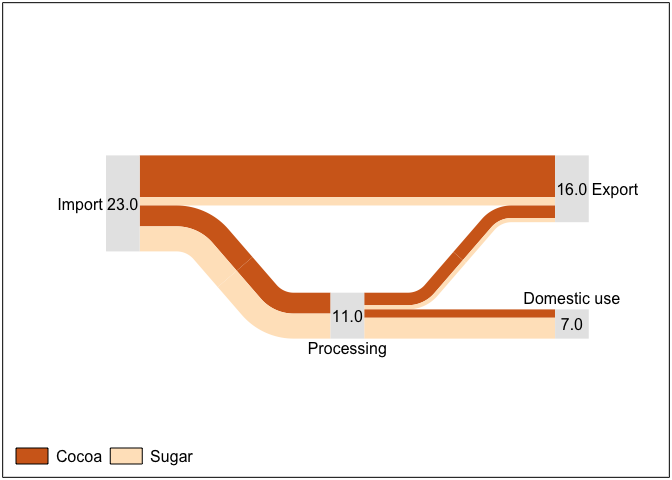Now the flows from Import to Export, and from Processing to Dometsic use, are rendered as a straight path.

Note that relative coordinates can refer to both absolute coordinates, or to another relative coordinate. This allows to set up diagrams with absolute coordinates for just one node, and all other nodes having coordinates relative to each other. This is illustrated in the next example

nodes <- tribble(
~ID,    ~label,          ~x, ~y, ~label_pos,
"imp",  "Import",         "0",       "0",    "left",
"exp",  "Export",         "proc+2", "imp",   "right",
"dom",  "Domestic use",   "exp",     "proc", "above",
"proc", "Processing",     "imp+2",   "imp-1", "below"
)
sankey(nodes, flows, colors, legend=TRUE)## 3.3 Node layout.

There are several options to control node layout. The option node_style (which must be a list) can be used to select a different type of node, e.g. "arrow", which uses a chevron-type arrow instead of the default box.

sankey(nodes, flows, colors, node_style=list(type="arrow"), legend=TRUE)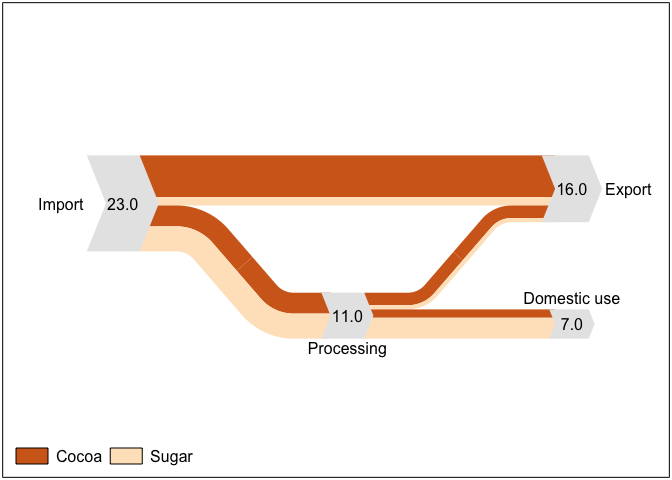Colors can be specified by also providing a list of graphical parameters, using the same format as base R’s grid package (i.e. the output of gpar()).

library(grid) # loads: gpar()
ns <- list(type="arrow", gp=gpar(fill="lightblue", col="white", lwd=4))
sankey(nodes, flows, colors, node_style=ns, legend=TRUE)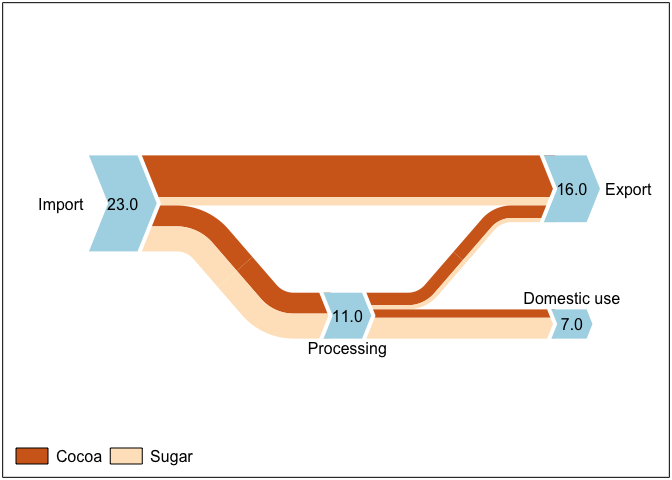## 3.4 Node magnitudes

The total amount of flow through a node (node magnitude') is plotted near the node. Node placement can be specified by using either a columnmag_posin the *nodes* data.frame, or by setting the optionmag_posin the call tosankey(), Valid options are:

• left, right, below,above – node magnitude is plotted left / right / etc. of the node.
• inside – centered within the node
• label – along with the node label.

note further that in the following example:

• The from field is not specified in for each individual flow. If an empty string is given, the previous value is re-used. This works similar for the to and what fields.
• In this example, only a single flow substance type is used, which is internally known as <any> (used in the Colors data.frame to refer to this flow type).
• An arrow node type, specified by setting node_type.
nodes <- tribble(
~ID,     ~label,       ~x,  ~y,       ~label_pos,
"in",    "Import",       0,  "1",    "left",
"proc",  "Processing",   2,  "0",    "below",
"out",   "Export",       4,  "in",   "right",
"use",   "Domestic use", 4,  "proc", "above"
)
flows <- tribble(
~from,   ~to,     ~quantity,
"in",    "out",    3.0,
"",      "proc",   2.0,
"proc",  "out",    1.5,
"",      "use",    0.5
)
colors <- tribble(
~substance,   ~color,
"<any>",      "cornflowerblue",
)

ns <- list(type="arrow", gp=gpar(fill="lightblue", col="white", lwd=4), mag_pos="label")
sankey(nodes, flows, colors, node_style=ns)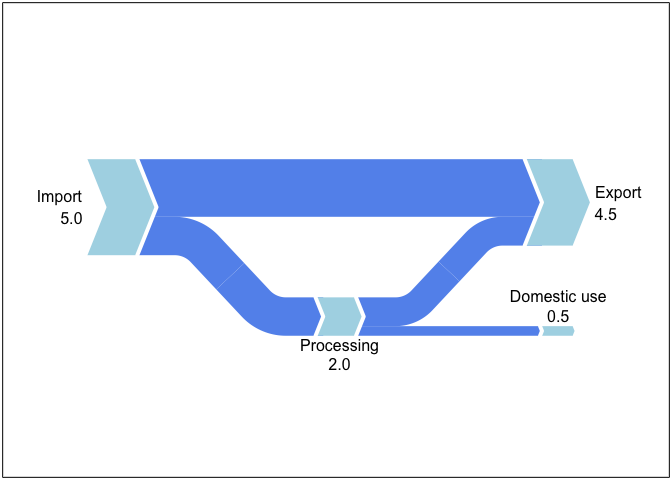## 3.5 Cycling.

The crux of true Sankey diagrams is in recycling; flows that feed pack into the process. This can be achieved by introducing additional nodes.

In the next example, the nodes R1, R2 and R3 are introduced (‘R’ for ‘recycling’). Note that

• label_pos for R1 is set to none to prevent a label
• the ID of R3 (in the nodes data.frame only!) is preceded by a dot to make it ‘hidden’ (similar to hidden files in *NIX operating systems)
• we used the option grill=TRUE in the call to sankey() to show a grid, which may be helpful when positioning the nodes.
nodes <- tribble(
~ID,     ~label,         ~x,   ~y,      ~dir,    ~label_pos,
"in",    "Import",       0,   "2",     "right", "left",
"proc",  "Processing",   4,   "0",     "right", "below",
"out",   "Export",       8,   "in",    "right", "right",
"use",   "Domestic use", 8,   "proc",  "right", "above",
"R1",    "",             7,   "-1.5",  "down",  "none",
"R2",    "Recycling",    4,   "-3",    "left",  "below",
".R3",   "",             1,   "-1.5",  "up",    "none"
)
flows <- tribble(
~from,    ~to,    ~quantity,
"in",     "out",   3.0,
"",       "proc",  2.0,
"proc",   "out",   1.5,
"",       "use",   0.5,
"proc",   "R1",    1.0,
"R1",     "R2",    1.0,
"R2",     "R3",   1.0,
"R3",    "proc",  1.0
)

colors <- tribble(
~substance, ~color,
"<any>",    "cornflowerblue",
)

ns <- list(type="arrow", gp=gpar(fill="red", col="white", lwd=3), mag_pos="label")
sankey(nodes, flows, colors, node_style=ns, grill=TRUE)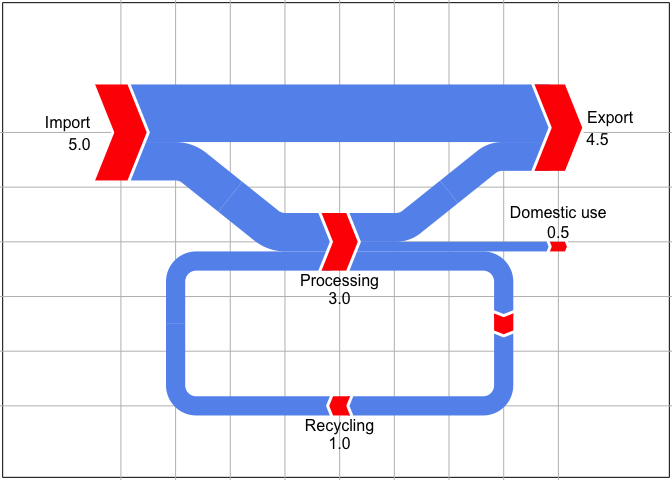# 4 Miscelaneous

## 4.2 Increasing margins

By default, a margin of 10% of the page size is used. This can be modified by setting the page_margin option. It can be either a scalar (margin), a 2-vector (x-margin, y-margin) or 4-vector (left,bottom,right,top).

The following example creates extra space near the bottom.

sankey(nodes, flows, colors, node_style=ns, copyright=copyright,
page_margin=c(0.1, 0.3, 0.1, 0.1))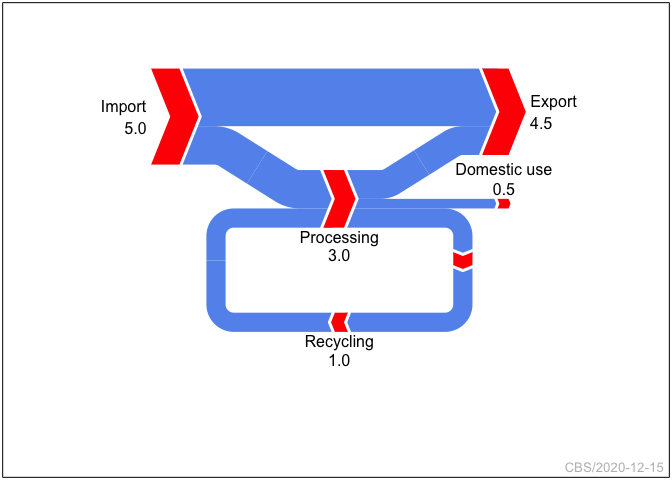## 4.3 Adding a stock node

Usually all internal nodes are in balance: output equals input, but sometimes this isn’t the case, e.g. in which a flow is added to some stock of unknown size, and another flow originates from this stock. This can be visualized by using a special stock’ node type, as the following example demonstrates:

nodes <- tribble(
~ID,     ~label,       ~x,   ~y,      ~dir,    ~label_pos,
"in",    "Import",      0,   "2",     "right", "left",
"stock", "Processing",  2,   "0",     "stock", "below",
"out",   "Export",      4,   "in",    "right", "right",
)
flows <- tribble(
~from,     ~to,      ~quantity,
"in",     "out",      1.5,
"in",     "stock",    2.0,
"stock",   "out",     1.0
)
colors <- tribble(
~substance, ~color,
"<any>",    "cornflowerblue",
)

ns <- list(type="arrow", gp=gpar(fill="red", col="white", lwd=4), mag_pos="label")
sankey(nodes, flows, colors,
node_style=ns,
page_margin=c(0.1, 0.2, 0.1, 0.1))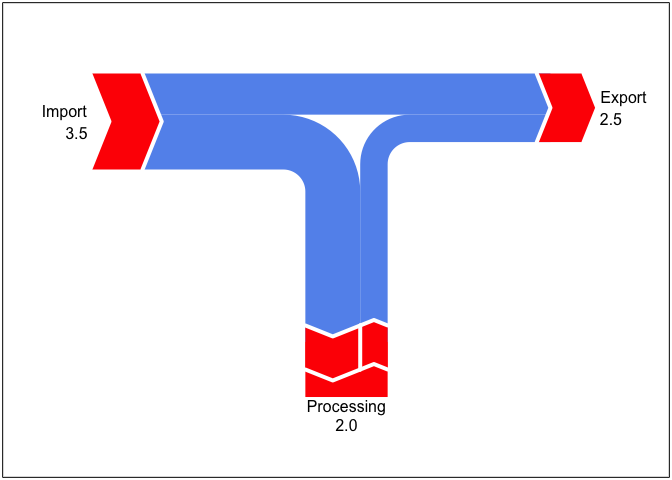## 4.4 Formatting the legend

nodes <- tribble(
~ID,  ~label,   ~x,   ~y,      ~dir,    ~label_pos,
"in",    "Input",  0,   "0",     "right", "left",
"out",   "Output", 4,   "in",    "right", "right",
)
flows <- tribble(
~from,     ~to,   ~quantity, ~substance,
"in",     "out",   1, "Oil",
"",       "",      1, "Gas",
"",       "",      1, "Biomass",
"",       "",      1, "Electricity",
"",       "",      1, "Solar",
"",       "",      1, "Hydrogen",
"",       "",      1, "Wind",
"",       "",      1, "Water",
"",       "",      1, "Nuclear",
)

ns <- list(type="arrow", gp=gpar(fill=gray(0.5), col="white", lwd=4), mag_pos="label")
sankey(nodes, flows, node_style=ns, legend=gpar(filesize=18, col="blue", ncols=2))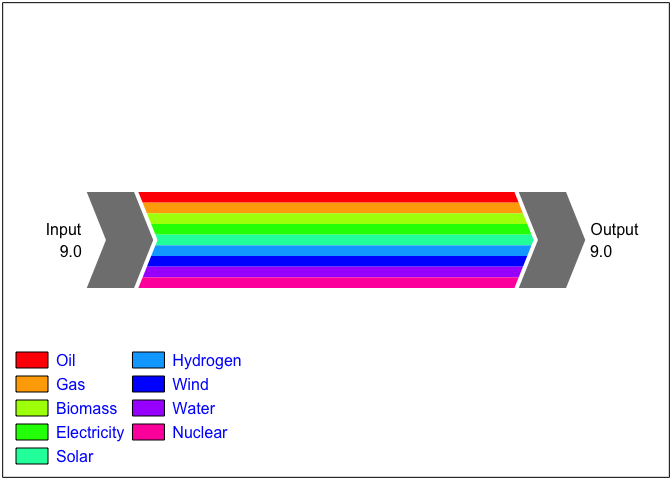## 4.5 Setting a title.

A title can be added to the Sankey diagram by setting the title option:

ns <- list(type="arrow", gp=gpar(fill=gray(0.5), col="white", lwd=4), mag_pos="label")
sankey(nodes, flows, node_style=ns, legend=gpar(filesize=18, col="blue", ncols=2),
page_margin=c(0.1, 0.1, 0.1, 0.2),
title="Panta Rhei")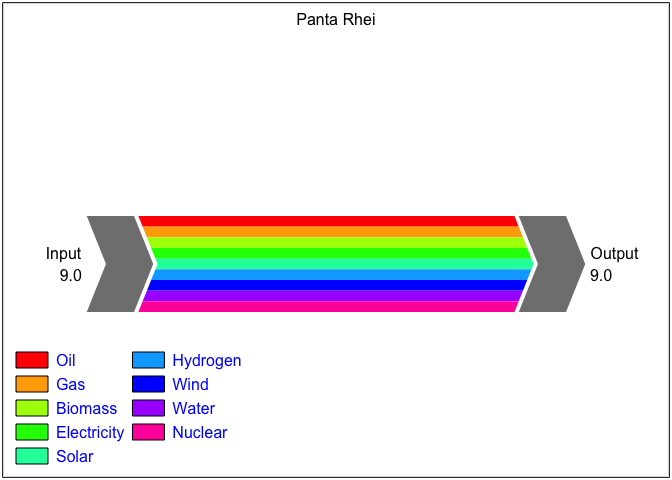Different font size, colors etc can be achieved by adding the output of a call to gpar as an attribute to the character string.

my_title <- "Panta Rhei"
attr(my_title, "gp") <- gpar(fontsize=24, fontface="bold", col="red")

sankey(nodes, flows, node_style=ns, legend=gpar(filesize=18, col="blue", ncols=2),
page_margin=c(0.1, 0.1, 0.1, 0.2),
title=my_title)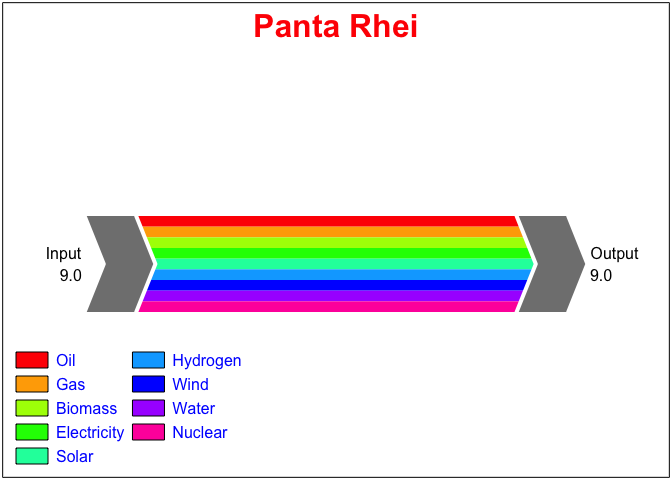for this end, the convenience function strformat() is available:

sankey(nodes, flows, node_style=ns, legend=gpar(filesize=18, col="blue", ncols=2),
page_margin=c(0.1, 0.1, 0.1, 0.2),
title=strformat("Panta Rhei", fontsize=18, col="blue"))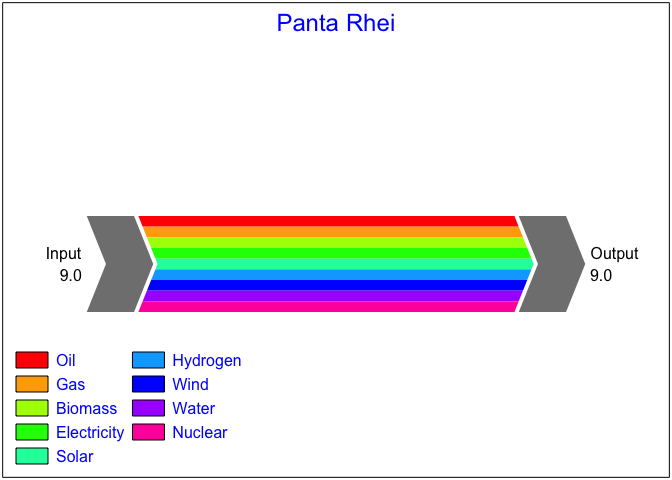## 4.6 Hardcopy outpout

Hardcopy output can be achieved by surrounding the call to sankey() by setting up a graphics device, e.g.

pdf("diagram.pdf", width=10, height=7) # Set up PDF device
sankey(nodes, flows, colors)           # plot diagram
dev.off()                              # close PDF device

Tip: If you want to have both visual and hardcopy output, you can put the call to sankey in a loop, exporting to the PDF only the second iteration.

In these examples, simple data sets where used. For real applications, data often is located elsewhere, e.g. in Excel spreadsheets. This is no problem; the various R libraries can be used to this end.

Example:

nodes   <- read_xlsx("my_sankey_data.xlsx", "nodes")
sankey(nodes, flows, colors)

Two helper functions are available to check the data sets

• check_consistency() which checks the consistency between the Nodes, Flows and Palette, for example by testing of all nodes referred to in the Flows table are defined in the Nodes table.
• check_balance() which checks if all nodes receive as much input as they generate output.
check_consistency(nodes, flows, colors)
check_balance(nodes, flows)

# 5 Final example,

For completeness, here is the example from the introduction. The data set is included with the package and can be loaded using

data(MFA) # Material Flow Account data

which load the MFA data as a list to wrap the nodes, flows, and color palette.

#> # A tibble: 16 x 7
#>    ID       label               label_pos label_align x         y          dir
#>    <chr>    <chr>               <chr>     <chr>       <chr>     <chr>      <chr>
#>  1 import   "Import (ex waste)" left      ""          -2        process+2  right
#>  2 imp_was… "Import (waste)"    left      ""          import    import-1.5 right
#>  3 extract  "Domestic extracti… left      ""          import    process-1  right
#>  4 process  "Materials\\nproce… below     "left"      3         0          right
#>  5 re_expo… "Re-export"         above     "left"      process   import     right
#>  6 material "Material use"      below     "right"     process+3 process-2  right
#>  7 energy   "Energetic use"     above     "left"      process+5 process-0… right
#>  8 stock    "Stocks"            right     ""          material… material-… stock
#>  9 short    "Short-lived produ… above     "left"      stock     material+… right
#> 10 .meat    ""                  none      ""          short-0.5 energy-0.2 right
#> 11 eol      "Waste"             below     ""          stock+2   material   right
#> 12 .R1      ""                  right     ""          eol+1.5   stock      down
#> 13 waste    "Losses"            right     ""          eol+2     energy     right
#> 14 export   "Export"            right     ""          waste     re_export  right
#> 15 R2       "Recycling"         below     "left"      material  stock-2    left
#> 16 .R3      ""                  none      ""          process-… material   up
#> # A tibble: 88 x 4
#>    from        to          substance quantity
#>    <chr>       <chr>       <chr>        <dbl>
#>  1 "import"    "re_export" Biomass      21.9
#>  2 ""          ""          Fossil       99.9
#>  3 ""          ""          Metals        9.00
#>  4 ""          ""          Mineral       5.69
#>  5 "re_export" "export"    Biomass      25.8
#>  6 ""          ""          Fossil      100.
#>  7 ""          ""          Metals       11.8
#>  8 ""          ""          Mineral       7.06
#>  9 "imp_waste" "process"   Biomass      11.1
#> 10 ""          ""          Fossil        1.34
#> # … with 78 more rows
#> # A tibble: 4 x 2
#>   substance color
#>   <chr>     <chr>
#> 1 Biomass   #206428
#> 2 Fossil    #ffd738
#> 3 Metals    #0d5f9d
#> 4 Mineral   #f7a01b
dblue <- "#00008B" # Dark blue

my_title <- "Material Flow Account"
attr(my_title, "gp") <- grid::gpar(fontsize=18, fontface="bold", col=dblue)

# node style
ns <- list(type="arrow",gp=gpar(fill=dblue, col="white", lwd=2),
length=0.7,
label_gp=gpar(col=dblue, fontsize=8),
mag_pos="label", mag_fmt="%.0f", mag_gp=gpar(fontsize=10,fontface="bold",col=dblue))

sankey(MFA$nodes, MFA$flows, MFA\$palette,
max_width=0.1, rmin=0.5,
node_style=ns,
page_margin=c(0.15, 0.05, 0.1, 0.1),
legend=TRUE, title=my_title,
copyright="Statistics Netherlands")